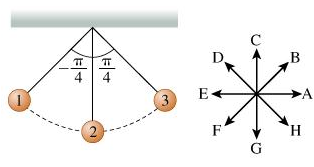# Problem: i) What is the direction of a when the pendulum is at position 1? Enter the letter of the arrow parallel to a_vec. ii) What is the direction of a at the moment the pendulum passes position 2? Enter the letter of the arrow that best approximates the direction of a. iii) What is the direction of a when the pendulum reaches position 3? Give the letter of the arrow that best approximates the direction of a. iv) As the pendulum approaches or recedes from which position(s) is the acceleration vector a almost parallel to the velocity vector v?

###### FREE Expert Solution

i)

The component of acceleration is gsinθ.

91% (80 ratings)###### Problem Detailsi) What is the direction of a when the pendulum is at position 1? Enter the letter of the arrow parallel to a_vec.

ii) What is the direction of a at the moment the pendulum passes position 2? Enter the letter of the arrow that best approximates the direction of a

iii) What is the direction of a when the pendulum reaches position 3? Give the letter of the arrow that best approximates the direction of a

iv) As the pendulum approaches or recedes from which position(s) is the acceleration vector a almost parallel to the velocity vector v?

Frequently Asked Questions

What scientific concept do you need to know in order to solve this problem?

Our tutors have indicated that to solve this problem you will need to apply the Intro to Oscillation concept. You can view video lessons to learn Intro to Oscillation. Or if you need more Intro to Oscillation practice, you can also practice Intro to Oscillation practice problems.

What professor is this problem relevant for?

Based on our data, we think this problem is relevant for Professor Lee's class at Southwestern College.